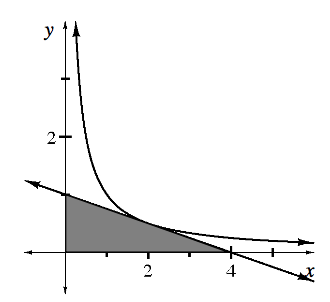### Home > APCALC > Chapter 6 > Lesson 6.2.2 > Problem6-77

6-77.

The graph of $y=\frac{1}{x}$ is shown at right. The tangent line at $(2,0.5)$, along with the coordinate axes, form a shaded triangle.

1. Write the equation of the tangent line.

Find $y^\prime$ and use point slope form to find the equation.

Point slope form: $y=m(x−x_1)+y_1$

2. Explain why the tangent line will always give an under approximation of the curve using the second derivative.

If the graph was concave down, would the tangent line be an over or under-approximation?

3. Calculate the area of the shaded triangle.

The base and height are given on the graph.

4. Calculate the area of the triangle formed when the tangent is instead placed at $(1,1)$.

Compute $y^\prime(1)$ and use point slope form again to find the equation.

5. Prove that the area of the shaded triangle formed by a tangent to $y=\frac{1}{x}$ is always the same, regardless of the point of tangency.

$\text{For a point }\left (a, \frac{1}{a} \right ),\text{use the result of } y'(a) \text{ and point slope form to find the equation of the tangent line.}$

The vertices of the triangle made by the tangent line are $(0,0)$, $(0,t(0))$, and ($x$-intercept of $\left(t(x),0\right)$, where $t(x)$ is the equation of the tangent line.

Calculate the area of the triangle using the vertices to determine the dimensions.